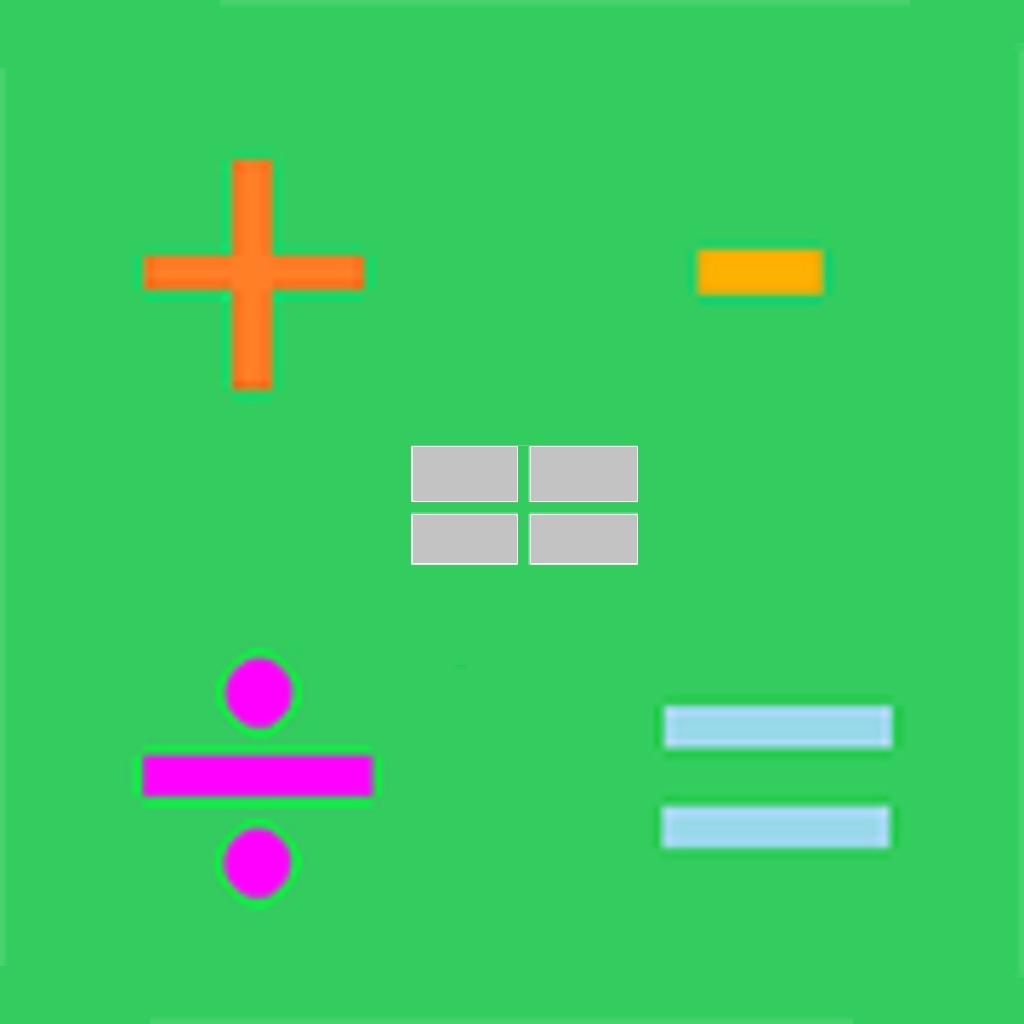Homesweet Learning helps students learn!High School Math Help!
MathJump! High School Math Enrichment is a teacher-led program to help high school students enrich their school Math curriculum and develop skills in problem solving, reasoning and proving, reflecting, selecting tools and computational strategies. Each class session is 1 hour. Other than the class sessions, students will also take a weekly online quiz and complete homework assignments. The program will review math concepts and techniques, as well as math questions that are challenging to the students.
High School Math Help! covers the following high school math topics:
• Grade 9: exponents, proportional reasoning, expressions and equations, data management, linear relations, properties of slope, relationship between equation of a relation and shape of its graph, optimal values of measurements of rectangles, perimeter, area, and volume, etc.